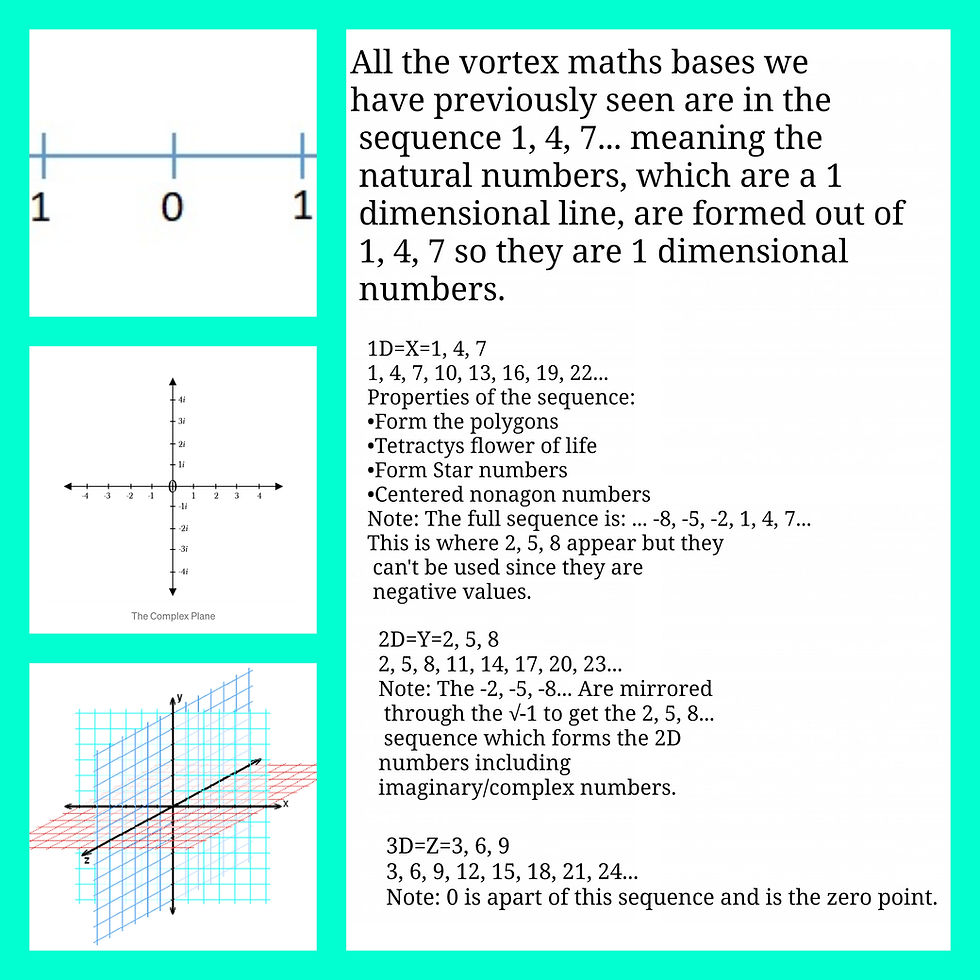top of page
Search

# Higher dimensional vortex mathsThe 3rd dimension is formed out of 1=147(1, 4, 7...), 2=258(2, 5, 8...), 3=369(3, 6, 9...) and therefor forms a new base 10 and 1, 4, 7... sequence which are all 3D vortex maths bases all contained within the 3D xyz grid. This means (the next three dimensions up to) the 6th dimension forms the 2, 5, 8... sequence and the 9th dimension forms the 3, 6, 9... sequence therefore the 9 dimensions form a new base 10 and 1, 4, 7... sequence meaning the 18th dimension forms the 2, 5, 8... sequence and the 27th dimension forms the 3, 6, 9... sequence and the 27 dimensions forms a new base 10 and 1, 4, 7... sequence and this repeats infinitely and as can be seen multiplying by 3 every time forming the powers of 3. At base infinity the infinite-gon(2D) or the infinite-hedron(3D), connecting the multiples of 3, and the doubling loop, which connects all other numbers that aren't multiples of 3, would become one also the infinite bases polygon would actually be a circle/sphere. The polygon/hedron fractal meaning at the infinite dimensions infinite base we would have an infinite dimensional fractaling sphere. The dimension of a fractal is in between dimensions, they are sub dimensions, and as we know each dimension contains its own numbers, such as 1D(numbers)=(Natural numbers) 2D(numbers)=(complex numbers), which are also sub dimensions of that dimension, all these sub dimensions fractally contain sub dimension. Therefore the infinite dimensions infinite base is the set which contains all sets, even itself through fractaling meaning each point/number is a (fractal) copy of the whole, it an infinitely growing (fractal) infinity. These dimensions are filled with points/numbers so they are a field of numbers, I named this field after imaginary numbers, it is called the imaginary field and at the unification of the doubling loop and multiples of 3 loop it becomes a field of 3, 6 and 9, the field of 9's, the 9 field. This field of 9 infinitely grows, an infinitely growing 9(9xen).